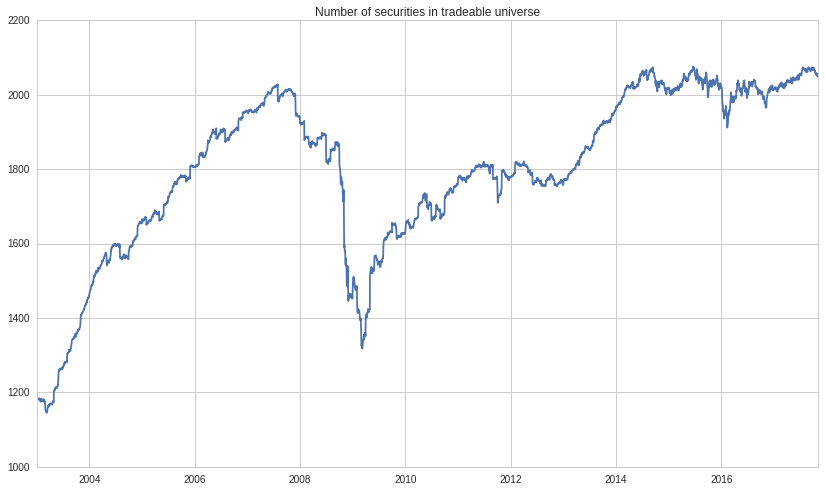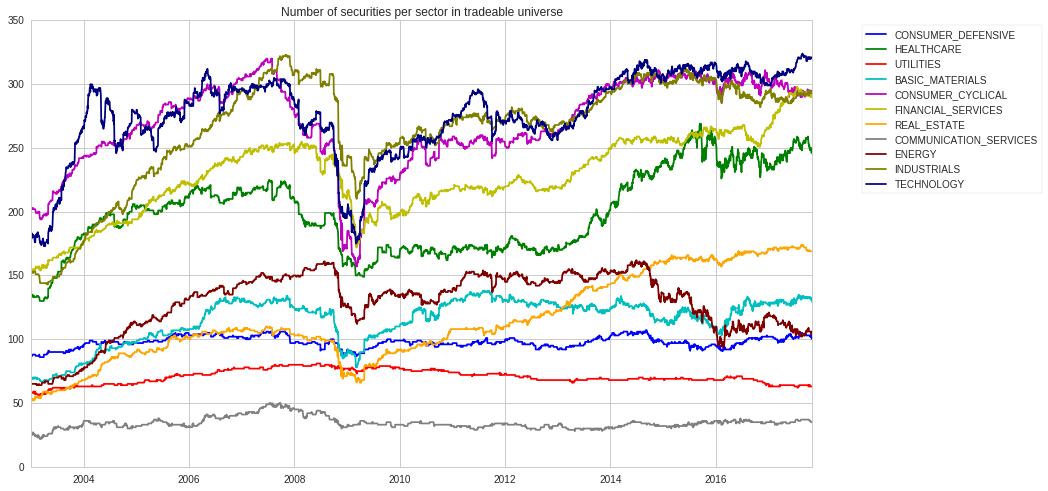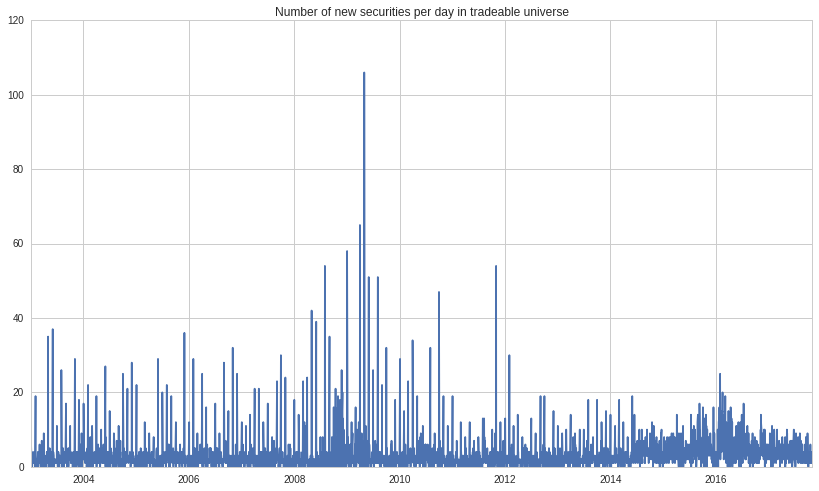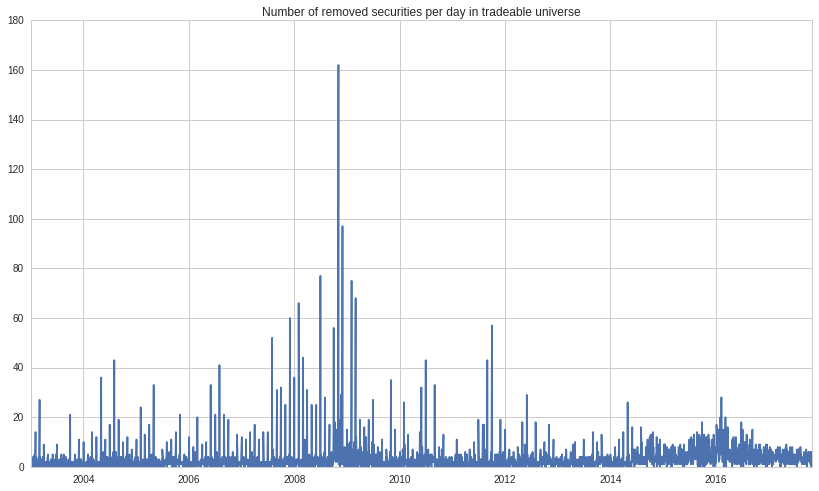Notebook

#### The new universe is updated daily rather than monthly.¶

In :
from quantopian.pipeline.data import Fundamentals
from quantopian.pipeline.data import morningstar
from quantopian.pipeline.factors import AverageDollarVolume
from quantopian.pipeline.factors.morningstar import MarketCap
from quantopian.pipeline.classifiers.morningstar import Sector
from quantopian.pipeline.data.builtin import USEquityPricing
from quantopian.pipeline import Pipeline
from quantopian.research import run_pipeline
import matplotlib.pyplot as plt
import numpy as np
import pandas as pd
from pandas import DataFrame as df
import time



## Pipeline Construction Using QTradableStocksUS as Screen¶

In :
# Function used to create a pipeline with the QTradableStocksUS as a screen. The pipeline contains common metrics as columns:
def make_pipeline():

average_day_dv_200 = AverageDollarVolume(window_length=200)
market_cap = Fundamentals.market_cap.latest
price = USEquityPricing.close.latest
volume = USEquityPricing.volume.latest
sector = Sector()

return Pipeline(
columns={
'AverageDollarVolume200': average_day_dv_200,
'MarketCap': market_cap,
'Price': price,
'Volume': volume,
'Sector': sector,
},
)

In :
# Pipeline is run over this time range and outputs a dataframe indexed by asset name:
START_DATE = '2003'
END_DATE = '2017-11-01'

start = time.time()
QTU_pipeline = run_pipeline(make_pipeline(), START_DATE, END_DATE, chunksize=252)
print 'Took %s seconds' % (time.time() - start)

Took 189.016684055 seconds


### Summary¶

In :
#Specific limits applied to the QTradableStocksUS:
#* Market cap over $500M #* daily dollar volume of$2.5m or more over the trailing 200 days
#* \$5 floor
#* 200 days of price and volume
#* Primary/Common share status of all assets
#* Exclusion of ADRs and LPs

In :
# Display constituent stocks of QTradableStocksUS:
QTU_pipeline

Out:
AverageDollarVolume200 MarketCap Price Sector Volume
2003-01-02 00:00:00+00:00 Equity(2 [ARNC]) 9.995053e+07 1.923248e+10 22.770 101 1895200.0
Equity(24 [AAPL]) 8.593046e+07 5.146413e+09 14.340 311 3292166.0
Equity(41 [ARCB]) 6.536824e+06 6.440801e+08 25.981 310 122175.0
Equity(60 [ABS]) 4.516864e+07 8.439904e+09 22.320 205 1399000.0
Equity(62 [ABT]) 1.978477e+08 2.810356e+10 39.950 206 7575500.0
Equity(64 [ABX]) 5.924553e+07 8.342951e+09 15.460 101 1612300.0
Equity(67 [ADSK]) 2.227898e+07 1.621620e+09 14.300 311 538104.0
Equity(76 [TAP]) 2.386964e+07 2.222835e+09 61.320 205 155400.0
Equity(85 [ACF]) 4.516536e+07 1.183216e+09 7.730 103 1759800.0
Equity(88 [ACI]) 5.976435e+06 1.130928e+09 21.510 101 153200.0
Equity(107 [ACV]) 1.239790e+07 2.534053e+09 50.430 205 153800.0
Equity(110 [ACXM]) 1.182046e+07 1.373709e+09 15.340 311 497486.0
Equity(114 [ADBE]) 1.057126e+08 5.937524e+09 24.800 311 2012664.0
Equity(122 [ADI]) 9.594235e+07 8.802856e+09 23.920 311 2352600.0
Equity(128 [ADM]) 1.741188e+07 8.019616e+09 12.400 205 992300.0
Equity(154 [AEM]) 1.087909e+07 1.242844e+09 14.800 101 473200.0
Equity(161 [AEP]) 7.759231e+07 9.311192e+09 27.250 207 1298300.0
Equity(168 [AET]) 5.934046e+07 6.233897e+09 41.110 206 800300.0
Equity(185 [AFL]) 4.874406e+07 1.554730e+10 30.180 103 1140100.0
Equity(197 [AGCO]) 1.440227e+07 1.657966e+09 22.020 310 579600.0
Equity(198 [AGE]) 1.484377e+07 2.632681e+09 32.930 103 319200.0
Equity(205 [AGN]) 7.118020e+07 7.457594e+09 57.650 206 947600.0
Equity(209 [AM]) 1.261291e+07 1.040538e+09 15.800 102 462700.0
Equity(216 [HES]) 4.948052e+07 4.910529e+09 55.020 309 559000.0
Equity(239 [AIG]) 3.861865e+08 1.264165e+11 57.660 103 4650500.0
Equity(266 [AJG]) 1.263031e+07 2.584611e+09 29.270 103 370000.0
Equity(272 [AL]) 4.016754e+07 8.483818e+09 29.510 101 422400.0
Equity(289 [MATX]) 2.967736e+06 5.604131e+08 25.790 310 136339.0
Equity(300 [ALK]) 5.344585e+06 5.753150e+08 21.610 310 158400.0
Equity(328 [ALTR]) 1.113936e+08 4.720455e+09 12.400 311 3631576.0
... ... ... ... ... ... ...
2017-11-01 00:00:00+00:00 Equity(50318 [APTI]) 4.254291e+06 9.689249e+08 24.250 311 2654399.0
Equity(50320 [ELF]) 1.427893e+07 9.768633e+08 21.190 205 456720.0
Equity(50325 [VVV]) 2.414149e+07 4.867057e+09 24.010 309 864983.0
Equity(50338 [NTNX]) 6.131955e+07 4.415894e+09 28.500 311 1949886.0
Equity(50350 [COUP]) 1.191831e+07 1.862745e+09 34.740 311 164693.0
Equity(50357 [ARCH]) 2.565638e+07 1.800499e+09 76.360 101 863173.0
Equity(50361 [ADSW]) 6.094025e+06 2.201865e+09 24.930 310 127302.0
Equity(50366 [CWH]) 1.244079e+07 3.618518e+09 42.020 102 684398.0
Equity(50368 [XOG]) 1.566365e+07 2.738124e+09 15.940 309 613147.0
Equity(50376 [CDEV]) 1.904420e+07 5.359314e+09 19.440 309 1191323.0
Equity(50382 [FTS]) 4.988358e+06 1.547612e+10 36.830 207 144552.0
Equity(50390 [ADNT]) 4.916479e+07 7.856170e+09 84.355 102 550550.0
Equity(50392 [YUMC]) 8.112479e+07 1.550551e+10 40.350 102 1236639.0
Equity(50399 [IRTC]) 8.487566e+06 1.158089e+09 50.950 206 202844.0
Equity(50403 [QCP]) 1.054689e+07 1.485005e+09 15.830 104 646879.0
Equity(50417 [QTNA]) 4.687968e+06 5.364207e+08 15.500 311 254107.0
Equity(50418 [BL]) 6.364629e+06 1.863739e+09 35.510 311 115036.0
Equity(50424 [GOLF]) 3.573180e+06 1.375128e+09 18.465 102 111451.0
Equity(50428 [AA]) 1.353879e+08 8.840396e+09 47.770 101 2356253.0
Equity(50430 [LW]) 3.217720e+07 7.454583e+09 51.000 205 322616.0
Equity(50444 [LEXE_A]) 8.965417e+06 2.664457e+09 46.070 311 223729.0
Equity(50499 [EVHC]) 7.184321e+07 5.147213e+09 42.600 206 2076525.0
Equity(50509 [ICHR]) 1.186581e+07 7.833634e+08 31.200 311 547858.0
Equity(50518 [ATH]) 4.774199e+07 1.062193e+10 52.110 103 858667.0
Equity(50526 [LGF_A]) 1.233745e+07 5.879658e+09 28.990 102 306808.0
Equity(50533 [CNDT]) 3.036826e+07 3.253128e+09 15.480 310 2132294.0
Equity(50534 [HGV]) 1.641287e+07 4.058684e+09 40.960 102 241375.0
Equity(50535 [PK]) 3.759613e+07 6.185261e+09 28.785 102 2279620.0
Equity(50537 [WRD]) 5.589958e+06 1.315770e+09 13.020 309 155817.0
Equity(50595 [BIVV]) 6.326426e+07 6.113292e+09 56.490 206 1366601.0

6721233 rows × 5 columns

In :
## The QTradableStocksUS universe generally contains a greater number of assets than previous iterations of the tradable universe.
## The resulting summary table displays the mean, std, min-max of daily median in addition to number of assets in this universe:
daily_constituent_count = QTU_pipeline.groupby(level=0).sum()
QTU_pipeline.groupby(level=0).median().describe()

Out:
AverageDollarVolume200 MarketCap Price Sector Volume
count 3.736000e+03 3.736000e+03 3736.000000 3736.0 3.736000e+03
mean 2.125409e+07 2.589276e+09 33.234946 206.0 6.687756e+05
std 4.180582e+06 4.800929e+08 4.908980 0.0 2.122386e+05
min 1.403000e+07 1.757724e+09 17.830000 206.0 1.318080e+05
25% 1.707031e+07 2.212792e+09 30.267500 206.0 5.314098e+05
50% 2.266303e+07 2.357533e+09 33.170000 206.0 6.153220e+05
75% 2.372152e+07 3.034414e+09 36.872500 206.0 7.526221e+05
max 3.344043e+07 3.683201e+09 43.690000 206.0 2.069636e+06

### Universe Size¶

In :
#Displays the number of assets in universe every day, which mirrors major economic events throughout the time period.
dates = QTU_pipeline.index.levels
grouped = QTU_pipeline.groupby(level=0).count()
num_securities = grouped['AverageDollarVolume200'].values
plt.plot(dates, num_securities)
plt.title('Number of securities in tradeable universe')

Out:
<matplotlib.text.Text at 0x7f96c34843d0>In :
#Number of assets in universe broken down by sector type:

colors = ['b', 'g', 'r', 'c', 'm', 'y', 'orange', 'gray', 'maroon', 'olive', 'navy']

for (sector, name), color in zip(Sector.SECTOR_NAMES.iteritems(), colors):
sector_result = QTU_pipeline.loc[QTU_pipeline['Sector'] == sector]
grouped = sector_result.groupby(level=0).count()
num_securities = grouped['AverageDollarVolume200'].values
plt.plot(dates, num_securities, label=name, color=color)

plt.legend(bbox_to_anchor=(1.3, 1))
plt.title('Number of securities per sector in tradeable universe')

Out:
<matplotlib.text.Text at 0x7f96c85a8a10>In :
#Number of assets added to universe is usually slightly greater than number of assets removed from universe.

assets_each_day = [set(df.loc[date].index) for date, df in QTU_pipeline.groupby(level=0)]
a = []
for i in range(1, len(assets_each_day)):
a.append(assets_each_day[i] - assets_each_day[i-1])

#Record the number of new assets to universe each day:
new_assets_each_day = pd.Series(a, dates[1:])
num_new_assets_each_day = new_assets_each_day.apply(lambda x: len(x))

b = []
for i in range(1, len(assets_each_day)):
b.append(assets_each_day[i-1] - assets_each_day[i])

#Record the number of assets removed from universe each day:
removed_assets_each_day = pd.Series(b, dates[1:])
num_removed_assets_each_day = removed_assets_each_day.apply(lambda x: len(x))

plt.plot(num_new_assets_each_day)
plt.title('Number of new securities per day in tradeable universe')

Out:
<matplotlib.text.Text at 0x7f96bf07a310>In [ ]:
plt.plot(num_removed_assets_each_day)
plt.title('Number of removed securities per day in tradeable universe')

Out[ ]:
<matplotlib.text.Text at 0x7f96c8e5af10>